Get inspired by the success stories of our students in IIT JAM MS, ISI  MStat, CMI MSc DS.  Learn More

# ISI MStat PSB 2008 Problem 10 | Hypothesis TestingThis is a really beautiful sample problem from ISI MStat PSB 2008 Problem 10. It is based on testing simple hypothesis. This problem teaches me how observation, makes life simple. Go for it!

## Problem- ISI MStat PSB 2008 Problem 10

Consider a population with three kinds of individuals labelled 1,2 and 3. Suppose the proportion of individuals of the three types are given by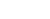, k=1,2,3 where 0<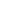<1.Let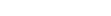be a random sample from this population. Find the most powerful test for testing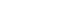versus. (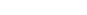).

### Prerequisites

Binomial Distribution.

Neyman-Pearson Lemma.

Test function and power function.

Hypothesis Testing.

## Solution :

This is a quite beautiful problem, only when you observe it closely. Here the distribution of X may seem non-trivial ( non-theoretical), but if one observes the distribution of Y=X-1 (say), instead of X , one will find that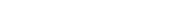.

so, now let, p= 1-, so, 0<p<1, and let,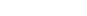and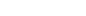.

and since ,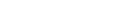, and our hypotheses, reduces to,versus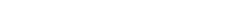.

so, under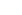, our joint pmf ( of Y=X-1), is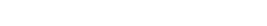; where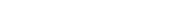and under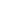, our joint pmf is,; whereSo, now we can use, widely used Neyman-Pearson Lemma , and end up with,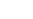=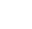=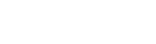=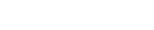.

now we define a test function,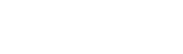. for some positive constant k.

Where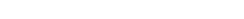so, our test rule is, we rejectif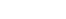, and we choose k such that the for a give level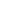,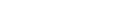, for a given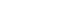,

with a power function ,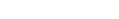. Can you find the more subtle condition when,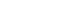? Try It!

## Food For Thought

Suppose,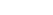, can you verify, that there for any constant c,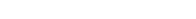. Can you generalize the situation, what kind distribution must X follow ?? Think over it, until we meet again !

## Subscribe to Cheenta at Youtube

This is a really beautiful sample problem from ISI MStat PSB 2008 Problem 10. It is based on testing simple hypothesis. This problem teaches me how observation, makes life simple. Go for it!

## Problem- ISI MStat PSB 2008 Problem 10

Consider a population with three kinds of individuals labelled 1,2 and 3. Suppose the proportion of individuals of the three types are given by, k=1,2,3 where 0<<1.Letbe a random sample from this population. Find the most powerful test for testingversus. ().

### Prerequisites

Binomial Distribution.

Neyman-Pearson Lemma.

Test function and power function.

Hypothesis Testing.

## Solution :

This is a quite beautiful problem, only when you observe it closely. Here the distribution of X may seem non-trivial ( non-theoretical), but if one observes the distribution of Y=X-1 (say), instead of X , one will find that.

so, now let, p= 1-, so, 0<p<1, and let,and.

and since ,, and our hypotheses, reduces to,versus.

so, under, our joint pmf ( of Y=X-1), is; whereand under, our joint pmf is,; whereSo, now we can use, widely used Neyman-Pearson Lemma , and end up with,===.

now we define a test function,. for some positive constant k.

Whereso, our test rule is, we rejectif, and we choose k such that the for a give level,, for a given,

with a power function ,. Can you find the more subtle condition when,? Try It!

## Food For Thought

Suppose,, can you verify, that there for any constant c,. Can you generalize the situation, what kind distribution must X follow ?? Think over it, until we meet again !

## Subscribe to Cheenta at Youtube

This site uses Akismet to reduce spam. Learn how your comment data is processed.

### Knowledge Partner